# Sets

## Introduction to Set Theory

### Collections of Fun!

Set Theory happens to be one of the easiest mathematical concepts taught in school. However, due to the immense stress laid on definitions over concepts, it appears to be a lot harder than it actually is.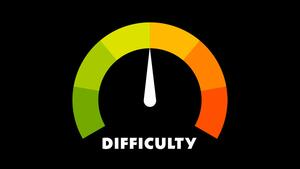## The Big Idea: What is a Set?

In simple words, a set is a collection of distinct objects, where the set itself is also considered an object.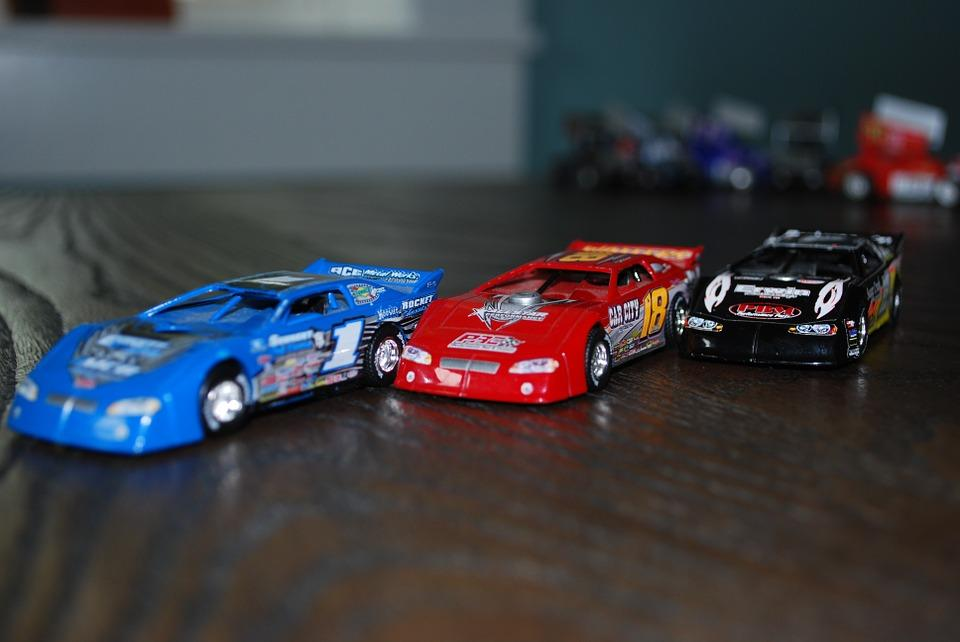A set is a collection of distinct objects, so suppose we defined a set of cars, it would look something like this:

Set A = {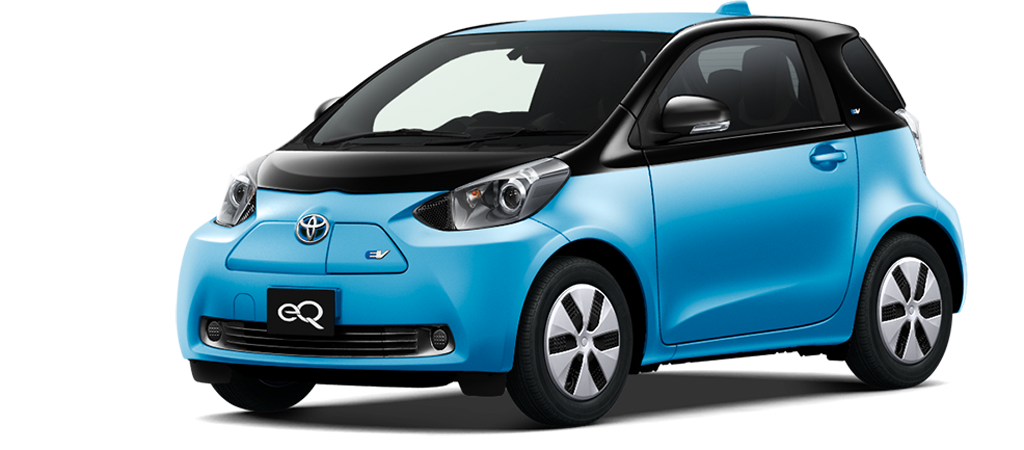,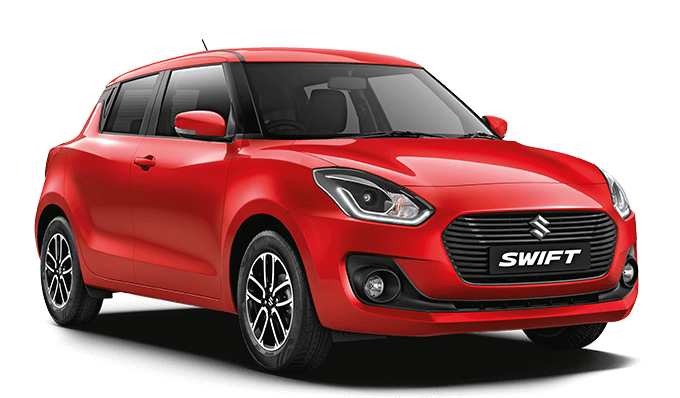,,          ,          ,          }

We would read the above set as ‘A’, a set of cars. Please note that each car is unique or distinct and no two cars are the same.

We use curly brackets { and } while writing a set. So, if you were writing a set B of numbers it would look something like this:

Set B = { 2, 5, 7, 12, 15, 18 }

## How do I understand?

### Describing Sets

There are two ways of defining a set. The first way is done by describing it in words. This is also known as the intensional definition.

For example, A is a set whose members are cars.
B is a set of six positive integers.

The second way of defining a set is as shown above with the pictures of the cars. It is known as the extensional definition, which is just a fancy way of enclosing the members of a set within curly brackets.

For example, Set C = {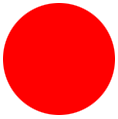,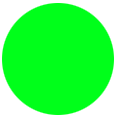,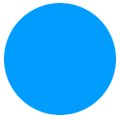}

That means C is a set whose members are the colours red, green and blue.

### Basic Set Operations

Suppose there was a toy box of toys, and X enjoyed playing with a few toys, and Y enjoyed playing with a few toys. For example:

Let X = {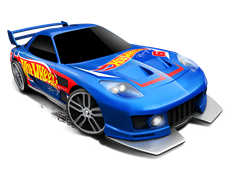,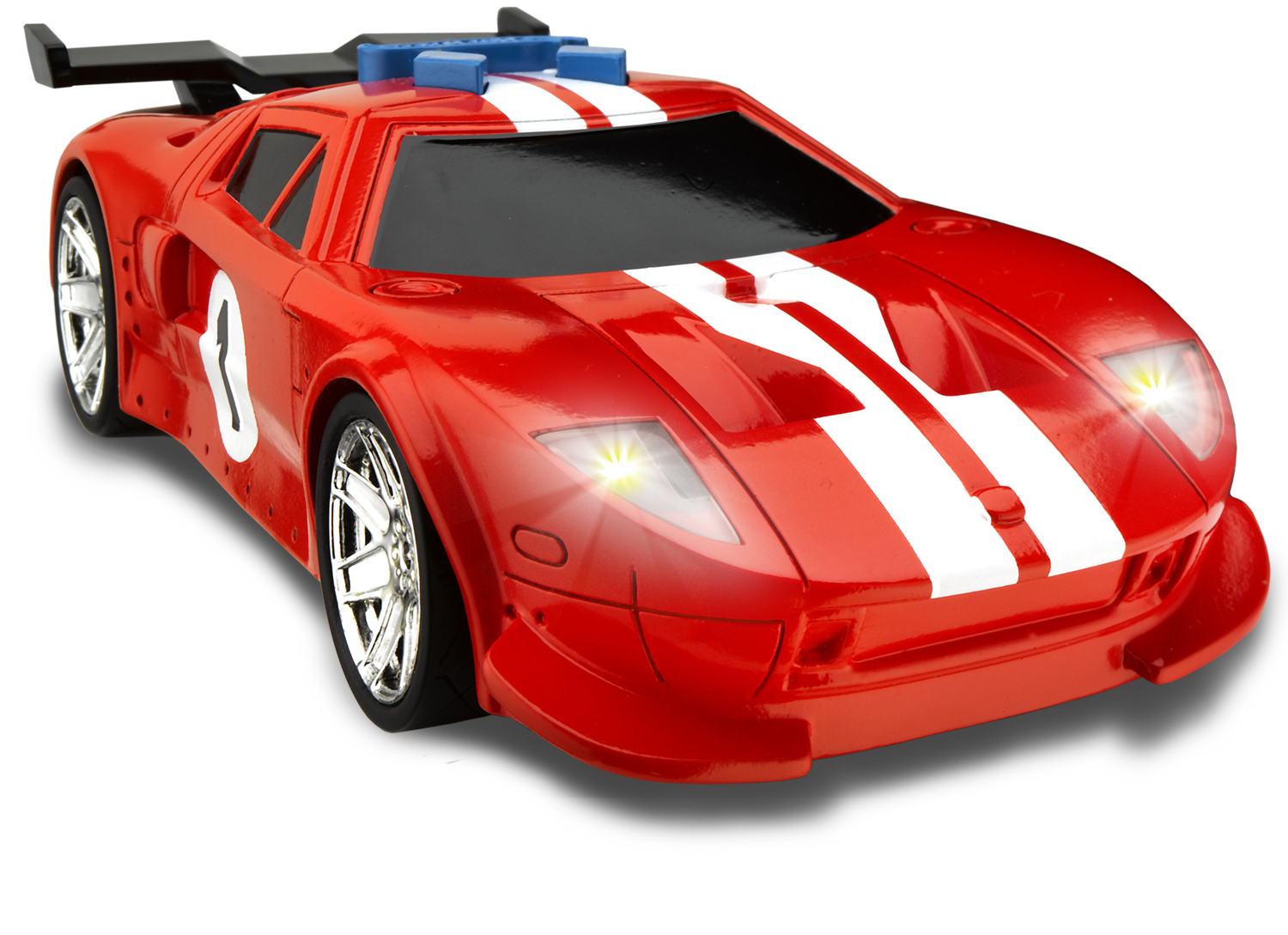,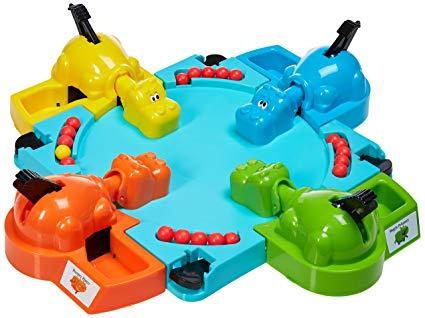,               }

And Y = {,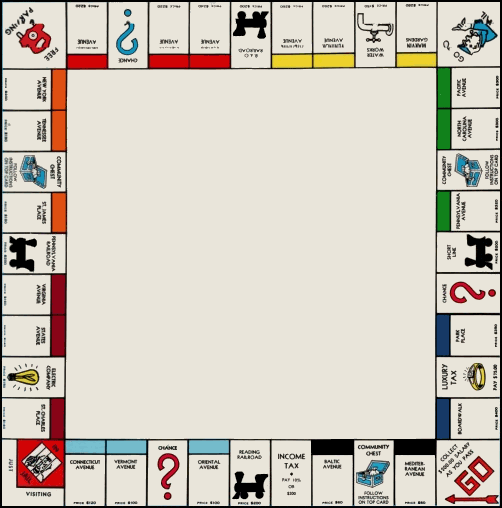,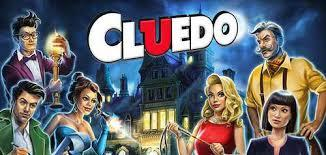,,               ,             }

So X plays with 2 Hot wheels cars and the Hungry Hippos board game. Y plays with Hungry Hippos, Monopoly, and the Cluedo board games along with the same Hot wheels car as X.

We would define the Union of X and Y as - objects that belong to set X or set Y

X ∪ Y = {,,,,,}

In simple words, the union of two sets X and Y have distinct objects that are present in set X OR Y.  While this can be confusing at times, it’s actually quite easy. The union of these two toy collections is basically a set which has all the toys in both these sets without repeating a toy twice - because there is only one toy of each kind. Since we have two toys that are common to both these collections, they are only counted once in the Union.

And now let’s define the Intersection of X and Y as - objects that belong to set X and set Y.

X ∩ Y ={,                       }

In simple words, the intersection of two sets X and Y have objects that are present in set X AND set Y. In this case, both X and Y have the dark blue car and Hungry Hippos in common, so they are the only objects in the intersection.

### Visualising Union and Intersection - Venn Diagrams

Another visually beautiful way of representing the union and intersection of two or more sets is by using Venn Diagrams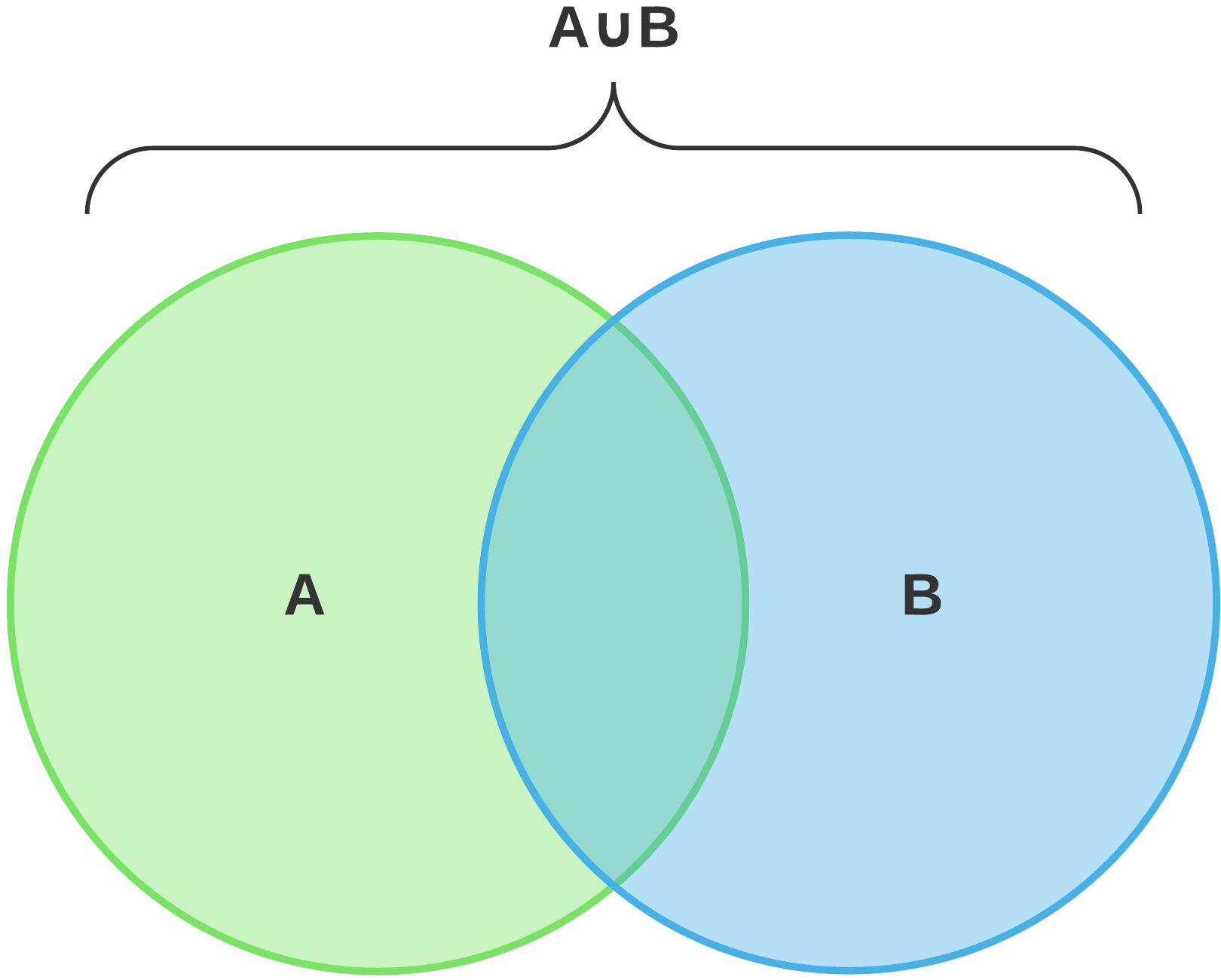Venn Diagrams make Set Theory a lot easier to understand. For example, if we took the sets X and Y from the previous example: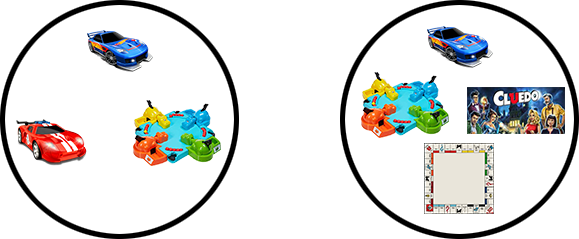X                                          Y

In venn diagrams it would look something like the below example:
So X ∪ Y or the union of X or Y would look like this: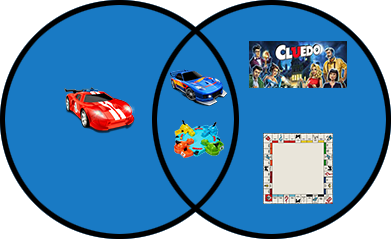And X ∩ Y or the intersection of X and Y would look like this: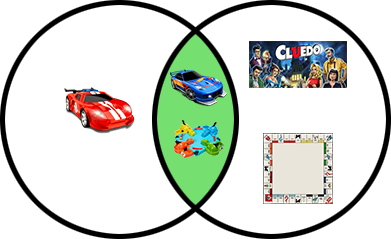Venn diagrams are popularly used to visualize problems in set theory to arrive at a solution. They are represented by overlapping circles.

### How is Set Theory important?

Set theory is an important foundational block for young mathematicians. It was largely developed to compute collections of objects and is used in various mathematical concepts like analysis, topology, abstract algebra and discrete mathematics.

Now that you’ve understood the basics of set theory, you are ready to take on more set theory operations like subsets, supersets, complements, relative complements and many more. Here are all the topics that are covered as part of the Cuemath curriculum across different grades:

## Quick Problems to get you started!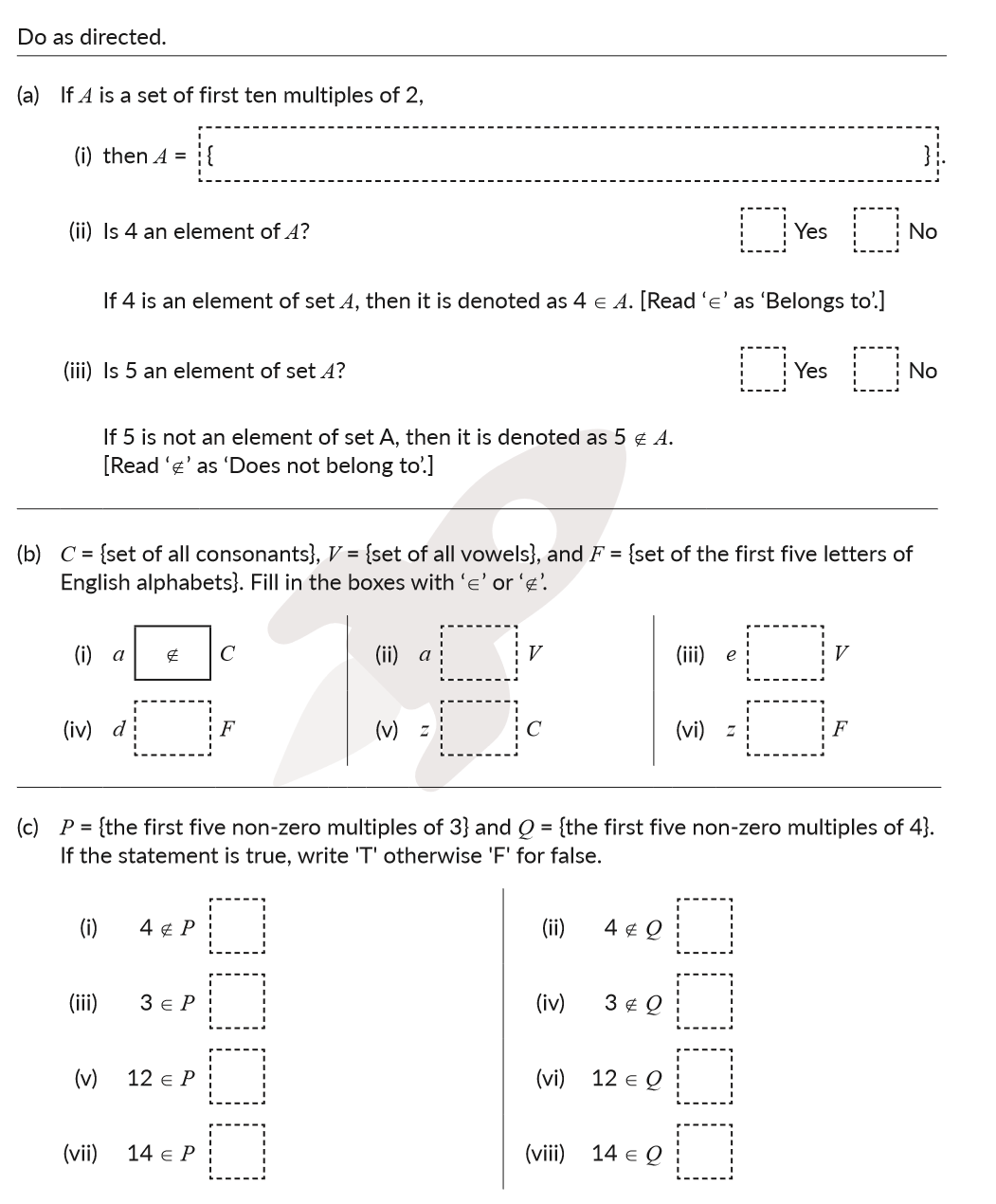## How do you teach Set Theory

Set theory as a mathematical concept is very easy for most children to understand. While some teachers prefer to use numbers to teach set theory, depending on the age of the classroom, numbers might be a little too dry. A popular yet effective way of teaching set theory is by assigning physical objects to the children in the classroom. Toys, tokens, blocks and other objects can be used to physically and visually create tangible collections of objects that can be used as analogies to drive home simple set theory operations. In younger classrooms, the student themselves can be part of the Venn diagrams drawn with chalk on the floor - creating interactive and fun learning sessions.

### Overcome common hurdles

Sometimes children do not fully understand the concept of distinct objects. This can be very confusing while explaining the union of two sets considering the Axiom of Union is an advanced concept not meant to be taught in high school. The Venn diagram solution is the best way to explain this concept. Children who can visualize mathematical concepts will take to set theory far easily than children who have difficulty visualizing. Remember to solve doubts if a child is struggling with a concept.

## Important Topics

Here are a few links that will take you through the journey that every Cuemath students undertakes in the pursuit of understanding Sets along with practice worksheets:

More Important Topics
Numbers
Algebra
Geometry
Measurement
Money
Data
Trigonometry
Calculus